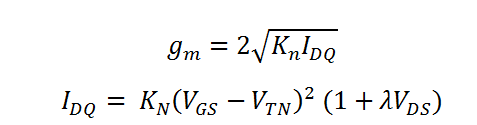# I-V relation and transconductance for saturation mode NMOS

This CalcTown calculator calculates the output current and transconductance in a NMOS in saturation mode.

Hence, the conditions 1. VGS>VTN  and 2. VDS>VGS-VTN are satisfied.

*Please enter 0 in the lambda and VDS field if the body effect is to be neglected.

** For potential divider bias circuits, gate-to-source voltage (VGS)=RG2VDD/(RG1+RG2)

V
V
uA/V2
V-1
V

#### Result

uA
uA/Vwhere Kn=(knW)/2L